# SAT Math : How to find the length of the hypotenuse of a 45/45/90 right isosceles triangle : Pythagorean Theorem

## Example Questions

### Example Question #1 : How To Find The Length Of The Hypotenuse Of A 45/45/90 Right Isosceles Triangle : Pythagorean Theorem

The length of the diagonal of a given square is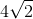. What is the square's area?Explanation:

If we divide the square into two triangles via its diagonal, then we know that the length of the diagonal is equal to the length of the triangles' hypotenuse.

We can use the Pythagorean Theorem to find the length of the two sides of one of our triangles.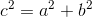Since we're dealing with a square, we know that the two sides of the square (which are the same as the two sides of one of our triangles) will be equal to one another. Therefore, we can say: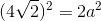Now, solve for the unknown: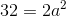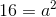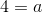This means that the length of the sides of our triangle, as well as the sides of our square, isTo find the area of the square, do the following: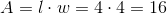.